# Clebsch-Gordan coefficients

This article presupposes knowledge of the quantum theory of angular momentum and angular momentum coupling.

In quantum mechanics, the Clebsch-Gordan coefficients (CG coefficients) are sets of numbers that arise in angular momentum coupling.

In mathematics, the CG coefficients appear in group representation theory, particularly of compact Lie groups. They arise in the explicit direct sum decomposition of the outer product of two irreducible representations (irreps) of a group G. In general the outer product representation (rep)—which is carried by a tensor product space—is reducible under G. Decomposition of the outer product rep into irreps of G requires a basis transformation of the tensor product space. The CG coefficients are the elements of the matrix of this basis transformation. In physics it is common to consider only orthonormal bases of the vector spaces involved, and then CG coefficients constitute a unitary matrix.

The name derives from the German mathematicians Alfred Clebsch (1833–1872) and Paul Gordan (1837–1912), who encountered an equivalent problem in invariant theory. An alternative name for the CG coefficients is Wigner coefficients, after Eugene Wigner (1902–1995).

The formulas below use Dirac's bra-ket notation, i.e., the quantity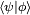$\langle \psi | \phi\rangle$ stands for a positive definite inner product between the elements ψ and φ of the same complex inner product space. We follow the physical convention$\langle c\psi | \phi \rangle = c^* \langle \psi | \phi \rangle$, where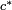$c^*\,$ is the complex conjugate of the complex number c.

## Angular momentum CG coefficients

Although Clebsch-Gordan coefficients can be defined for arbitrary groups, we restrict our attention in this article to the groups associated with space and spin angular momentum, namely the groups SO(3) and SU(2). In that case CG coefficients can be defined as the expansion coefficients of total angular momentum eigenstates in an uncoupled tensor product basis. See the article angular momentum for the definition of angular momentum operators and their eigenstates. Since the definition of CG coefficients needs the definition of tensor products of angular momentum eigenstates, this will be given first.

From the formal definition recursion relations for the Clebsch-Gordan coefficients can be found. In order to settle the numerical values for the coefficients, a phase convention must be adopted. Below the Condon and Shortley phase convention is chosen.

## Tensor product space

Let V1 be the 2j1 + 1 dimensional vector space spanned by the eigenstates of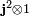$\mathbf{j}^2\otimes 1$ and$j_z\otimes 1$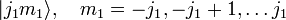$|j_1 m_1\rangle,\quad m_1=-j_1,-j_1+1,\ldots j_1$

and V2 the 2j2 + 1 dimensional vector space spanned by the eigenstates of$1\otimes\mathbf{j}^2$ and$1\otimes j_z$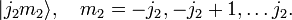$|j_2 m_2\rangle,\quad m_2=-j_2,-j_2+1,\ldots j_2.$

The tensor product of these spaces,$V_{12}\equiv V_1\otimes V_2$, has a (2j1 + 1)(2j2 + 1) dimensional uncoupled basis$|j_1 m_1\rangle|j_2 m_2\rangle \equiv |j_1 m_1\rangle \otimes |j_2 m_2\rangle, \quad m_1=-j_1,\ldots j_1, \quad m_2=-j_2,\ldots j_2.$

Angular momentum operators acting on V12 can be defined by$(j_i \otimes 1)|j_1 m_1\rangle|j_2 m_2\rangle \equiv (j_i|j_1m_1\rangle) \otimes |j_2m_2\rangle, \quad i = x,y,z,$

and$(1 \otimes j_i) |j_1 m_1\rangle|j_2 m_2\rangle) \equiv |j_1m_1\rangle \otimes j_i|j_2m_2\rangle,\quad i = x,y,z.$

Total angular momentum operators are defined by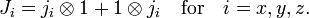$J_i = j_i \otimes 1 + 1 \otimes j_i\quad\mathrm{for}\quad i = x,y,z.$

The total angular momentum operators satisfy the commutation relations$[J_k,J_l] = i \sum_{m=x,y,z} \epsilon_{klm}J_m \,$

showing that J is indeed an angular momentum operator. The quantity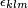$\epsilon_{klm}$ is the Levi-Civita symbol. Hence total angular momentum eigenstates exist$\mathbf{J}^2 |(j_1j_2)JM\rangle = J(J+1) |(j_1j_2)JM\rangle$$J_z |(j_1j_2)JM\rangle = M |(j_1j_2)JM\rangle,\quad \mathrm{for}\quad M=-J,\ldots,J$

It can be derived—see, e.g., this article—that J must satisfy the triangular condition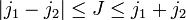$|j_1-j_2| \leq J \leq j_1+j_2$

The total number of total angular momentum eigenstates is equal to the dimension of V12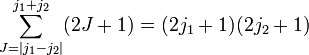$\sum_{J=|j_1-j_2|}^{j_1+j_2} (2J+1) = (2j_1+1)(2j_2+1)$

The total angular momentum states form an orthonormal basis of V12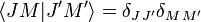$\langle J M | J' M' \rangle = \delta_{J\, J'}\delta_{M\,M'}$

## Formal definition of Clebsch-Gordan coefficients

The total angular momentum states can be expanded in the uncoupled basis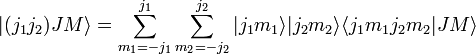$|(j_1j_2)JM\rangle = \sum_{m_1=-j_1}^{j_1} \sum_{m_2=-j_2}^{j_2} |j_1m_1\rangle|j_2m_2\rangle \langle j_1m_1j_2m_2|JM\rangle$

The expansion coefficients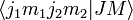$\langle j_1m_1j_2m_2|JM\rangle$ are called Clebsch-Gordan coefficients.

Applying the operator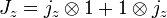$J_z = j_z \otimes 1 + 1 \otimes j_z$

to both sides of the defining equation shows that the Clebsch-Gordan coefficients can only be nonzero when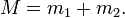$M = m_1 + m_2.\,$

## Recursion relations

Applying the total angular momentum raising and lowering operators, see e.g., this article,$J_\pm = j_\pm \otimes 1 + 1 \otimes j_\pm$

to the left hand side of the defining equation gives$J_\pm|(j_1j_2)JM\rangle = C_\pm(J,M) |(j_1j_2)JM\pm 1\rangle = C_\pm(J,M)\sum_{m_1m_2}|j_1m_1\rangle|j_2m_2\rangle \langle j_1 m_1 j_2 m_2|J M\pm 1\rangle.$

Applying the same operators to the right hand side gives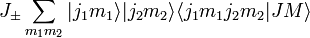$J_\pm \sum_{m_1m_2} |j_1m_1\rangle|j_2m_2\rangle \langle j_1m_1j_2m_2|JM\rangle$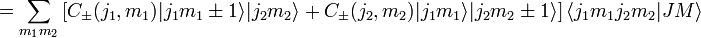$=\sum_{m_1m_2}\left[ C_\pm(j_1,m_1)|j_1 m_1\pm 1\rangle |j_2m_2\rangle +C_\pm(j_2,m_2)|j_1 m_1\rangle |j_2 m_2\pm 1\rangle \right] \langle j_1 m_1 j_2 m_2|J M\rangle$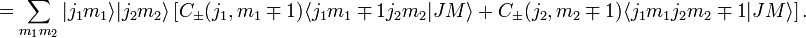$= \sum_{m_1m_2} |j_1m_1\rangle|j_2m_2\rangle \left[ C_\pm(j_1,m_1\mp 1) \langle j_1 {m_1\mp 1} j_2 m_2|J M\rangle +C_\pm(j_2,m_2\mp 1) \langle j_1 m_1 j_2 {m_2\mp 1}|J M\rangle \right].$

Combining these results gives recursion relations for the Clebsch-Gordan coefficients$C_\pm(J,M) \langle j_1 m_1 j_2 m_2|J M\pm 1\rangle = C_\pm(j_1,m_1\mp 1) \langle j_1 {m_1\mp 1} j_2 m_2|J M\rangle + C_\pm(j_2,m_2\mp 1) \langle j_1 m_1 j_2 {m_2\mp 1}|J M\rangle.$

Taking the upper sign with M = J gives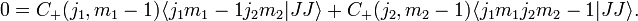$0 = C_+(j_1,m_1-1) \langle j_1 {m_1-1} j_2 m_2|J J\rangle + C_+(j_2,m_2-1) \langle j_1 m_1 j_2 m_2-1|J J\rangle.$

In the Condon and Shortley phase convention the coefficient$\langle j_1 j_1 j_2 J-j_1|J J\rangle$ is taken real and positive. With the last equation all other Clebsch-Gordan coefficients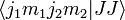$\langle j_1 m_1 j_2 m_2|J J\rangle$ can be found. The normalization is fixed by the requirement that the sum of the squares, which corresponds to the norm of the state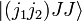$|(j_1j_2)JJ\rangle$ must be one.

The lower sign in the recursion relation can be used to find all the Clebsch-Gordan coefficients with M = J − 1. Repeated use of that equation gives all coefficients.

This procedure to find the Clebsch-Gordan coefficients shows that they are all real (in the Condon and Shortley phase convention).

## Explicit expression

The first derivation of an algebraic formula for CG coefficients was given by Wigner in his famous 1931 book. The following expression for the CG coefficients is due to Van der Waerden (1932) and is the most symmetric one of the various existing forms, see, e.g., Biedenharn and Louck (1981) for a derivation,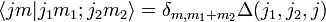$\langle jm | j_1 m_1 ;j_2 m_2 \rangle = \delta_{m,m_1+m_2} \Delta (j_1 ,j_2 ,j)$$\times \sum_t (-1)^t {\textstyle \frac{ \left[(2j+1) (j_1 +m_1 )! (j_1 -m_1)! (j_2 +m_2 )! (j_2 -m_2 )! (j+m)! (j-m)! \right]^{\frac{1}{2}}}{t! (j_1 +j_2 -j-t)! (j_1 -m_1 -t)! (j_2 +m_2 -t)! (j-j_2 +m_1 +t)! (j-j_1 -m_2 +t)!} }$

where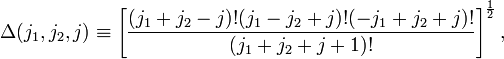$\Delta (j_1 ,j_2 ,j) \equiv \left[ \frac{ (j_1 +j_2 -j)! (j_1 -j_2 +j)! (-j_1 +j_2 +j)!} { (j_1 +j_2 +j+1)!}\right]^{{\frac{1}{2}}},$

and the sum runs over all values of t which do not lead to negative factorials.

## Orthogonality relations

These are most clearly written down by introducing the alternative notation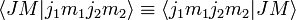$\langle J M|j_1 m_1 j_2 m_2\rangle \equiv \langle j_1 m_1 j_2 m_2|J M \rangle$

The first orthogonality relation is$\sum_{J=|j_1-j_2|}^{j_1+j_2} \sum_{M=-J}^{J} \langle j_1 m_1 j_2 m_2|J M \rangle \langle J M|j_1 m_1' j_2 m_2'\rangle = \delta_{m_1,m_1'}\delta_{m_2,m_2'}$

and the second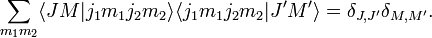$\sum_{m_1m_2} \langle J M|j_1 m_1 j_2 m_2\rangle \langle j_1 m_1 j_2 m_2|J' M' \rangle = \delta_{J,J'}\delta_{M,M'}.$

## Special cases

For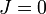$J=0\,$ the Clebsch-Gordan coefficients are given by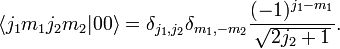$\langle j_1 m_1 j_2 m_2 | 0 0 \rangle = \delta_{j_1,j_2}\delta_{m_1,-m_2} \frac{(-1)^{j_1-m_1}}{\sqrt{2j_2+1}}.$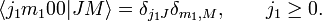$\langle j_1 m_1 0 0 | J M \rangle = \delta_{j_1 J}\delta_{m_1,M}, \qquad j_1 \ge 0.$

For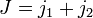$J=j_1+j_2\,$ and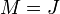$M=J\,$ we have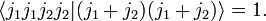$\langle j_1 j_1 j_2 j_2 | (j_1+j_2) (j_1+j_2) \rangle = 1.$

## Symmetry properties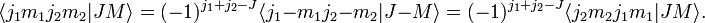$\langle j_1 m_1 j_2 m_2|J M \rangle = (-1)^{j_1+j_2-J} \langle j_1 {-m_1} j_2 {-m_2}|J {-M}\rangle = (-1)^{j_1+j_2-J} \langle j_2 m_2 j_1 m_1|J M \rangle.$

## Relation to 3-jm symbols

Clebsch-Gordan coefficients are related to 3-jm symbols which have more convenient symmetry relations.$\langle j_1 m_1 j_2 m_2 | j_3 m_3 \rangle = (-1)^{j_1-j_2+m_3}\sqrt{2j_3+1} \begin{pmatrix} j_1 & j_2 & j_3\\ m_1 & m_2 & -m_3 \end{pmatrix}.$